Related Articles

# Widest Path Problem | Practical application of Dijkstra’s Algorithm

• Difficulty Level : Hard
• Last Updated : 01 Sep, 2021

It is highly recommended to read Dijkstra’s algorithm using the Priority Queue first.
Widest Path Problem is a problem of finding a path between two vertices of the graph maximizing the weight of the minimum-weight edge in the path. See the below image to get the idea of the problem: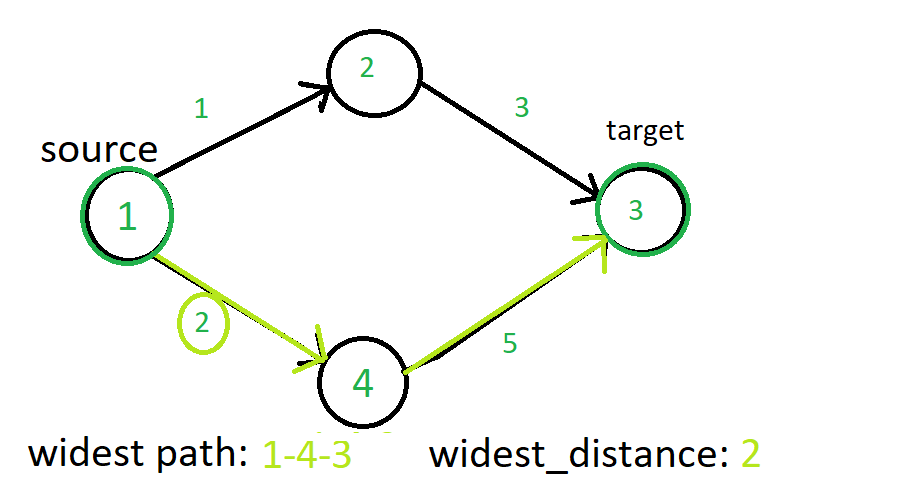Practical Application Example:
This problem is a famous variant of Dijkstra’s algorithm. In the practical application, this problem can be seen as a graph with routers as its vertices and edges represent bandwidth between two vertices. Now if we want to find the maximum bandwidth path between two places in the internet connection, then this problem can be solved by this algorithm.
How to solve this problem?
We are going to solve this problem by using the priority queue ((|E|+|V|)log|V|) implementation of the Dijkstra’s algorithm with a slight change.
We solve this problem by just replacing the condition of relaxation in Dijkstra’s algorithm by:

max(min(widest_dist[u], weight(u, v)), widest_dist[v])

where u is the source vertex for v. v is the current vertex we are checking the condition.
This algorithm runs for both directed and undirected graph.
See the series of images below to get the idea about the problem and the algorithm:
The values over the edges represents weights of directed edges.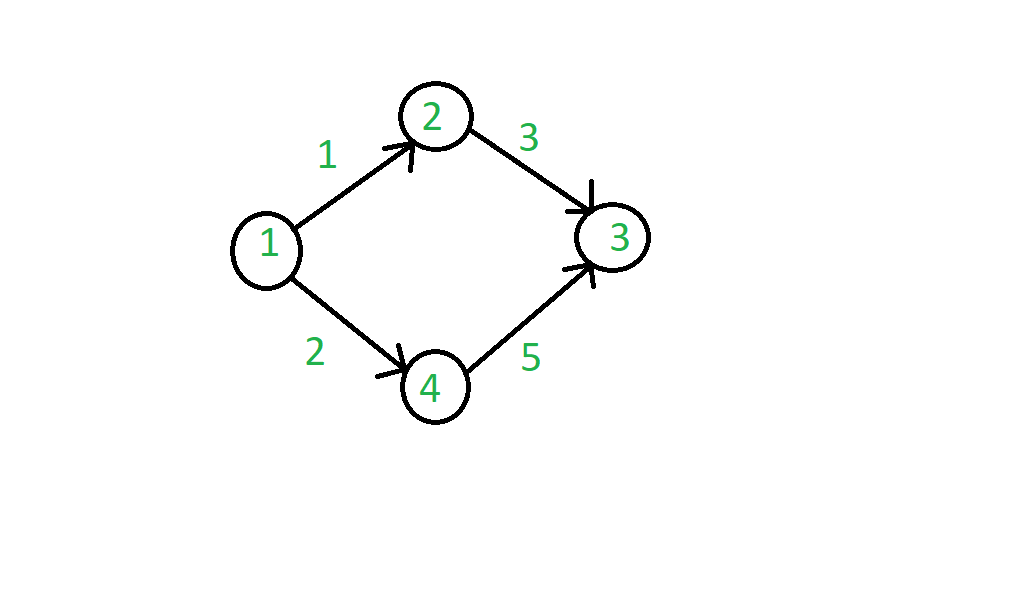We will start from source vertex and then travel all the vertex connected to it and add in priority queue according to relaxation condition.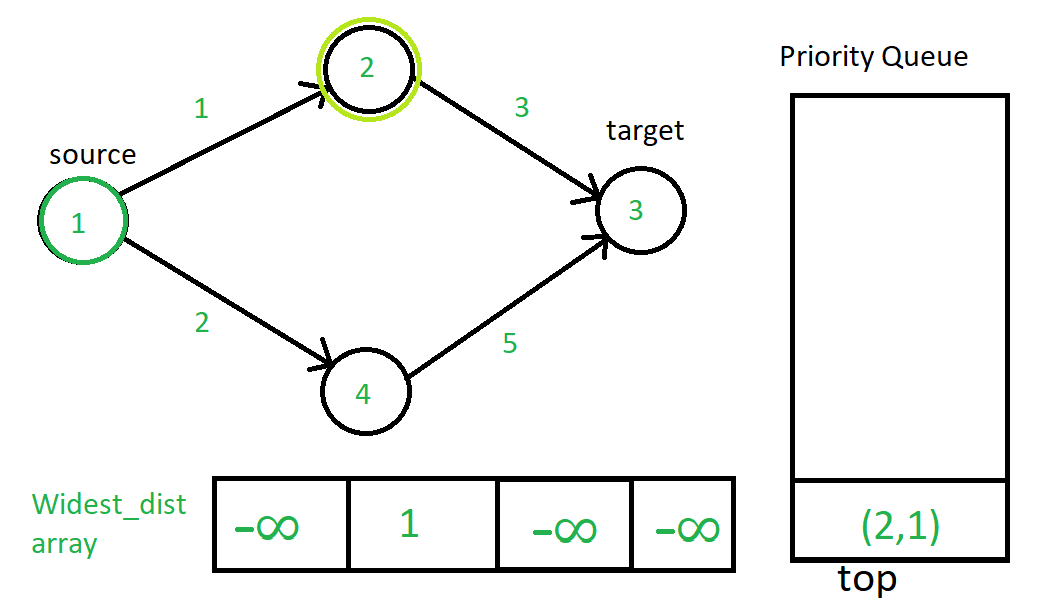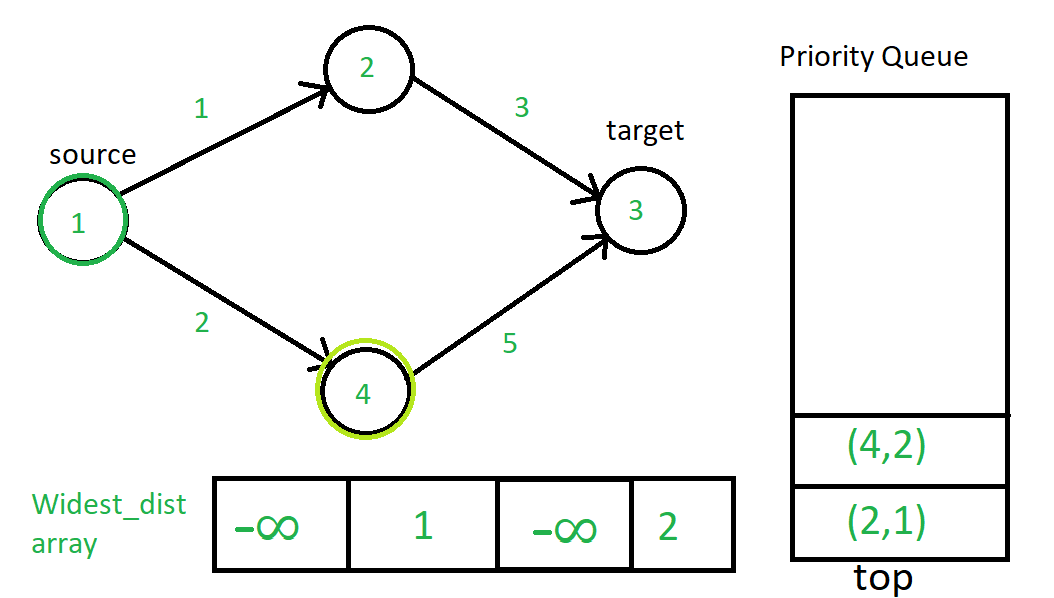Now (2, 1) will pop up and 2 will be the current source vertex.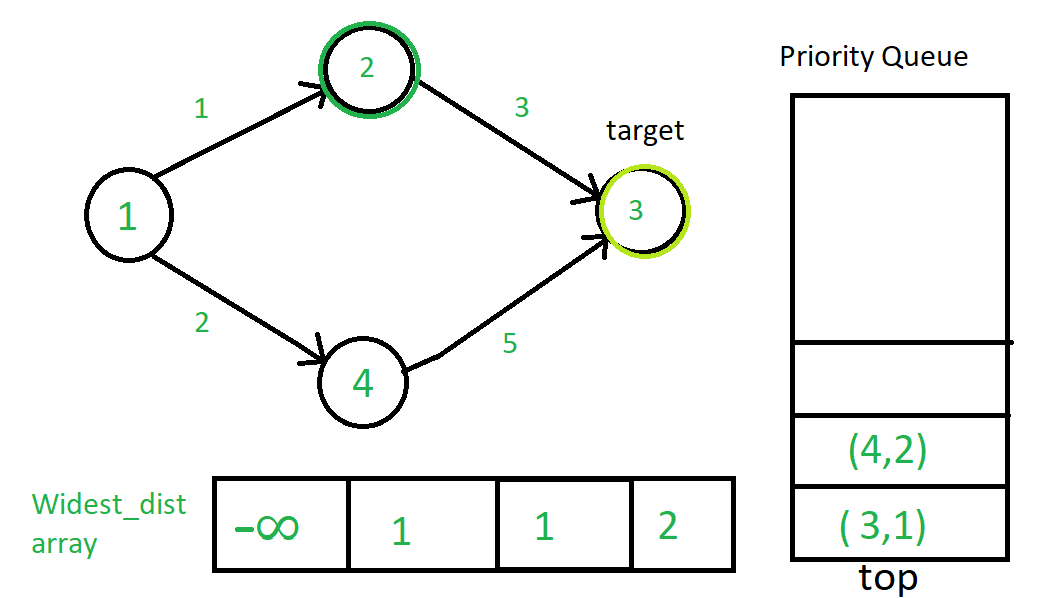Now (3, 1) will pop from the queue. But as 3 does not have any connected vertex through directed edge nothing will happen. So (4, 2) will pop next.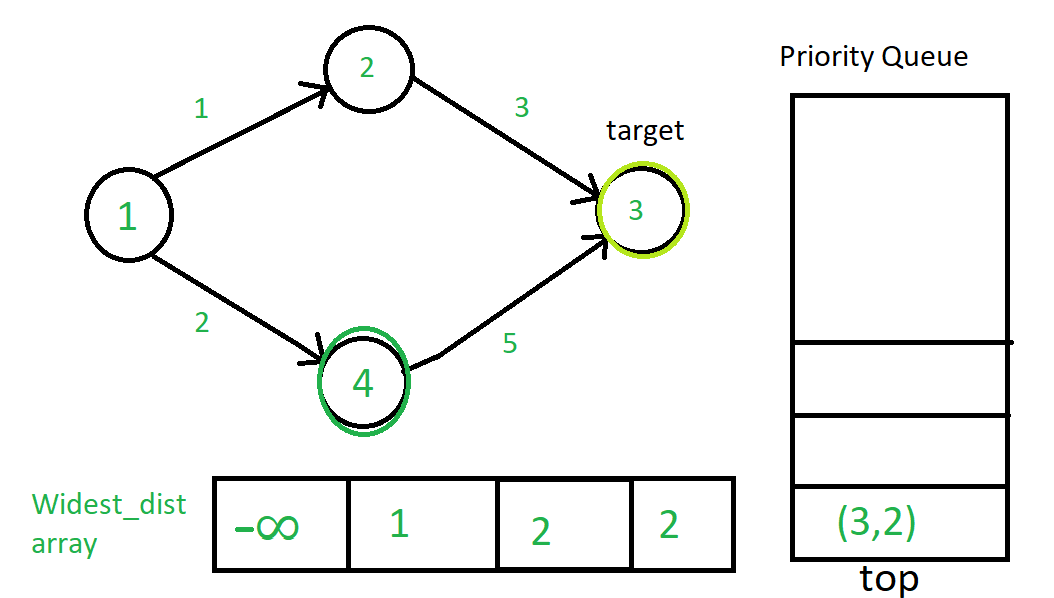Finally the algorithm stops, as there is no more elements in priority queue.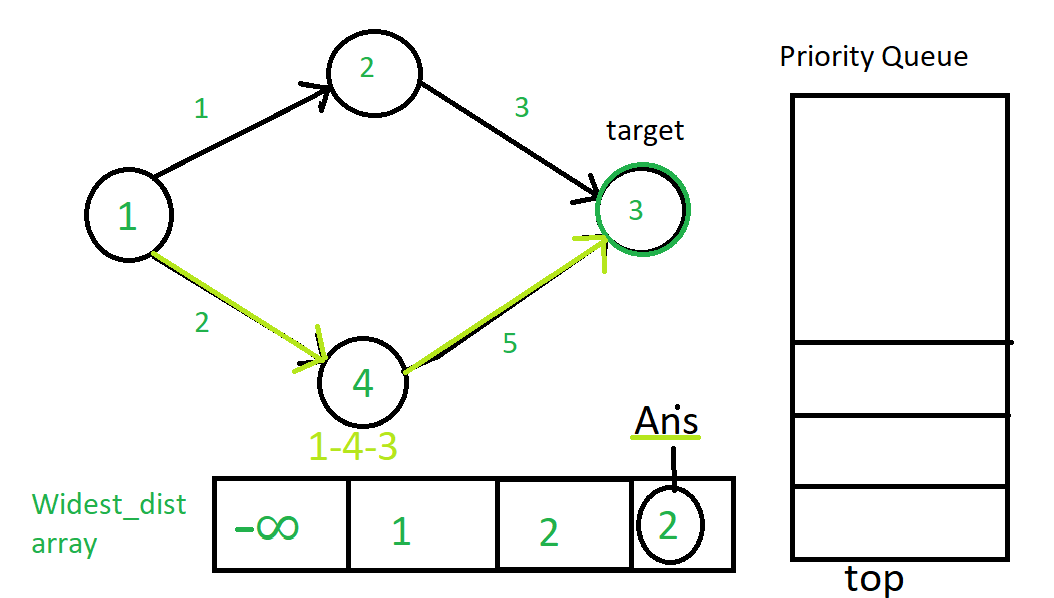The path with the maximum value of widest distance is 1-4-3 which has the maximum bottle-neck value of 2. So we end up getting widest distance of 2 to reach the target vertex 3.
Below is the implementation of the above approach:

## CPP

 `// C++ implementation of the approach` `#include ` `using` `namespace` `std;`   `// Function to print the required path` `void` `printpath(vector<``int``>& parent, ``int` `vertex, ``int` `target)` `{` `    ``if` `(vertex == 0) {` `        ``return``;` `    ``}`   `    ``printpath(parent, parent[vertex], target);`   `    ``cout << vertex << (vertex == target ? ``"\n"` `: ``"--"``);` `}`   `// Function to return the maximum weight` `// in the widest path of the given graph` `int` `widest_path_problem(vector > >& Graph,` `                        ``int` `src, ``int` `target)` `{` `    ``// To keep track of widest distance` `    ``vector<``int``> widest(Graph.size(), INT_MIN);`   `    ``// To get the path at the end of the algorithm` `    ``vector<``int``> parent(Graph.size(), 0);`   `    ``// Use of Minimum Priority Queue to keep track minimum` `    ``// widest distance vertex so far in the algorithm` `    ``priority_queue, vector >,` `                   ``greater > >` `        ``container;`   `    ``container.push(make_pair(0, src));`   `    ``widest[src] = INT_MAX;`   `    ``while` `(container.empty() == ``false``) {` `        ``pair<``int``, ``int``> temp = container.top();`   `        ``int` `current_src = temp.second;`   `        ``container.pop();`   `        ``for` `(``auto` `vertex : Graph[current_src]) {`   `            ``// Finding the widest distance to the vertex` `            ``// using current_source vertex's widest distance` `            ``// and its widest distance so far` `            ``int` `distance = max(widest[vertex.second],` `                               ``min(widest[current_src], vertex.first));`   `            ``// Relaxation of edge and adding into Priority Queue` `            ``if` `(distance > widest[vertex.second]) {`   `                ``// Updating bottle-neck distance` `                ``widest[vertex.second] = distance;`   `                ``// To keep track of parent` `                ``parent[vertex.second] = current_src;`   `                ``// Adding the relaxed edge in the priority queue` `                ``container.push(make_pair(distance, vertex.second));` `            ``}` `        ``}` `    ``}`   `    ``printpath(parent, target, target);`   `    ``return` `widest[target];` `}`   `// Driver code` `int` `main()` `{`   `    ``// Graph representation` `    ``vector > > graph;`   `    ``int` `no_vertices = 4;`   `    ``graph.assign(no_vertices + 1, vector >());`   `    ``// Adding edges to graph`   `    ``// Resulting graph` `    ``// 1--2` `    ``// |  |` `    ``// 4--3`   `    ``// Note that order in pair is (distance, vertex)` `    ``graph.push_back(make_pair(1, 2));` `    ``graph.push_back(make_pair(2, 4));` `    ``graph.push_back(make_pair(3, 3));` `    ``graph.push_back(make_pair(5, 3));`   `    ``cout << widest_path_problem(graph, 1, 3);`   `    ``return` `0;` `}`

## Python3

 `# Python3 implementation of the approach`   `# Function to print required path` `def` `printpath(parent, vertex, target):` `    `  `    ``# global parent` `    ``if` `(vertex ``=``=` `0``):` `        ``return` `    ``printpath(parent, parent[vertex], target)` `    ``print``(vertex ,end``=``"\n"` `if` `(vertex ``=``=` `target) ``else` `"--"``)`   `# Function to return the maximum weight` `# in the widest path of the given graph` `def` `widest_path_problem(Graph, src, target):` `    `  `    ``# To keep track of widest distance` `    ``widest ``=` `[``-``10``*``*``9``]``*``(``len``(Graph))`   `    ``# To get the path at the end of the algorithm` `    ``parent ``=` `[``0``]``*``len``(Graph)`   `    ``# Use of Minimum Priority Queue to keep track minimum` `    ``# widest distance vertex so far in the algorithm` `    ``container ``=` `[]` `    ``container.append((``0``, src))` `    ``widest[src] ``=` `10``*``*``9` `    ``container ``=` `sorted``(container)` `    ``while` `(``len``(container)>``0``):` `        ``temp ``=` `container[``-``1``]` `        ``current_src ``=` `temp[``1``]` `        ``del` `container[``-``1``]` `        ``for` `vertex ``in` `Graph[current_src]:`   `            ``# Finding the widest distance to the vertex` `            ``# using current_source vertex's widest distance` `            ``# and its widest distance so far` `            ``distance ``=` `max``(widest[vertex[``1``]], ` `                           ``min``(widest[current_src], vertex[``0``]))`   `            ``# Relaxation of edge and adding into Priority Queue` `            ``if` `(distance > widest[vertex[``1``]]):`   `                ``# Updating bottle-neck distance` `                ``widest[vertex[``1``]] ``=` `distance`   `                ``# To keep track of parent` `                ``parent[vertex[``1``]] ``=` `current_src`   `                ``# Adding the relaxed edge in the priority queue` `                ``container.append((distance, vertex[``1``]))` `                ``container ``=` `sorted``(container)` `    ``printpath(parent, target, target)` `    ``return` `widest[target]`   `# Driver code` `if` `__name__ ``=``=` `'__main__'``:`   `    ``# Graph representation` `    ``graph ``=` `[[] ``for` `i ``in` `range``(``5``)]` `    ``no_vertices ``=` `4` `    ``# Adding edges to graph`   `    ``# Resulting graph` `    ``#1--2` `    ``#|  |` `    ``#4--3`   `    ``# Note that order in pair is (distance, vertex)` `    ``graph[``1``].append((``1``, ``2``))` `    ``graph[``1``].append((``2``, ``4``))` `    ``graph[``2``].append((``3``, ``3``))` `    ``graph[``4``].append((``5``, ``3``))`   `    ``print``(widest_path_problem(graph, ``1``, ``3``))`   `# This code is contributed by mohit kumar 29`

Output:

```1--4--3
2```

Time Complexity: O(E * logV), Where E is the total number of edges and V is the total number of vertices in the graph.
Auxiliary Space: O(V).

Attention reader! Don’t stop learning now. Get hold of all the important DSA concepts with the DSA Self Paced Course at a student-friendly price and become industry ready.  To complete your preparation from learning a language to DS Algo and many more,  please refer Complete Interview Preparation Course.

In case you wish to attend live classes with experts, please refer DSA Live Classes for Working Professionals and Competitive Programming Live for Students.

My Personal Notes arrow_drop_up
Recommended Articles
Page :